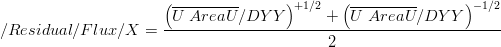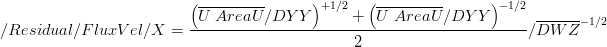Residual

(diff) ← Older revision | Latest revision (diff) | Newer revision → (diff)

MohidWater may calculate time averages on certain hydrodynamic parameters at the end of each run. The variables whose time averages are calculated are the Velocity, Flux, FluxVel and the Waterlevel and they appear in the hdf5 file under the group /Residual. In particular the residual flux is the mean between the cell's faces of the time averaged face flux divided by the face width.

Definitions

• Velocity - time averaged velocity during the period of the simulation
• Flux - time averaged specific flow in m2/s which is the flow in m3/s divided by the width in meters of the grid cell) during the period of the simulation
• FluxVel - time averaged specific flow divided by the average grid cell thickness in m/s which is the specific flow (m2/s) divided by the average thickness of the grid cell in meters during the period of the simulation
• Waterlevel - time averaged water elevation during the period of the simulation

Setup

Simply turn on the residual option in the Hydrodynamic.dat file:

RESIDUAL             : 1

Formulas$/Residual/Flux/X = \frac{\left( \overline{U\;AreaU} / DYY \right)^{+1/2} + \left( \overline{U\;AreaU} / DYY \right)^{-1/2}}{2}$$/Residual/FluxVel/X = \frac{\left( \overline{U\;AreaU} / DYY \right)^{+1/2} + \left( \overline{U\;AreaU} / DYY \right)^{-1/2}}{2}/\overline{DWZ}^{-1/2}$